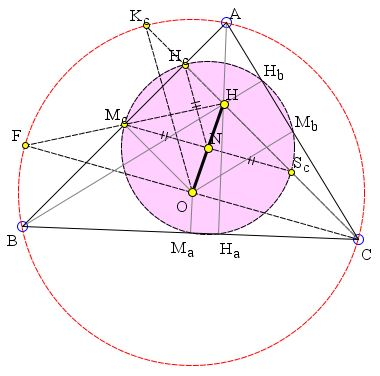# Nine Point Circle: an Elementary Proof

In any triangle, the orthocenter and the circumcenter are collinear with center N of circle that passes through the midpoints of the sides, the feet of the altitudes, and the midpoints of the segments joining the orthocenter with the vertices. The radius of the circle is half the circumradius. In addition, HN = NO

The circle is known as the 9-point circle and its center as the 9-point center.

### If you are reading this, your browser is not set to run Java applets. Try IE11 or Safari and declare the site https://www.cut-the-knot.org as trusted in the Java setup.What if applet does not run?

DiscussionThe proof of the existence of the 9-point circle illustrated by the applet is due to Vladimir Nikolin, an elementary school teacher from Serbia.

The key to the proof is the observation that the antipodes of the vertices in the circumcircle along with the orthocenter and two vertices form parallelograms whose diagonals intersect at the midpoints of the sides of the triangle. We already used this observation twice: when proving that reflections of the orthocenter in the side lines of a triangle lie on its circumcenter, and in a simple proof of the existence of the Euler line.Let Ha, Ma, Sa be the foot of the altitude from A, the midpoint of the side BC (opposite A) and the midpoint of AH. Points Hb, Mb, Sb, Hc, Mc, Sc are defined similarly. AD, BE, and CF are diameters of the circumcircle of ΔABC.

Let's focus on vertex C and its antipode F, so that CF is a diameter of the circumcircle. As we know, HD passes through Mc and is divided by Mc into halves: HMc = DMc.

By definition, also HSc = CSc, implying that McSc is a midline of ΔCHD so that McSc||CD. Let N be the intersection of McSc and HO: HN = NO. Now, in ΔDHO, EMc = OD/2. If R is the circumradius of ΔABC then OD = R and we have EMc = R/2. In ΔCHO, EHc = R/2.

As was already shown, HHc = FHc. It follows that in ΔHOF NMc is the midline parallel to OF = R.

To summarize, NSc = NMc = NF = R/2. Similarly we show that the remaining six points also lie on the circle with center N and radius R/2.

N is another famous point (along with the orthocenter, centroid, and circumcenter) that lies on the Euler line. The 9-point circle is also known as Euler's circle and sometimes Feuerbach's circle.

(Note: Another simple argument can be found elsewhere at the site.)### Nine Point Circle